Learn Chinese Characters Lesson 4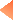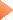This is the fourth of five free lessons about Chinese characters' strokes.

Lesson 3:

Order Of Strokes Examples

This lesson contains examples of "regular" Chinese characters. Next one will contain some more "complicated" examples.

ORDER OF STROKES
Example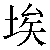Sequence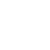ExampleSequence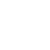Example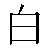Sequence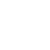Example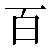Sequence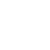Example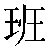Sequence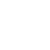Example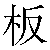Sequence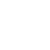Example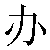Sequence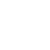Example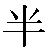Sequence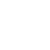ExampleSequenceExample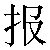Sequence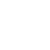Example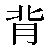Sequence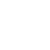Example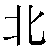Sequence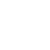Example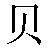Sequence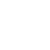Example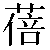Sequence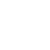Example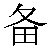Sequence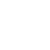Example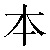Sequence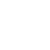Example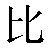Sequence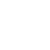Example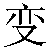SequenceExample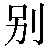Sequence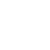ExampleSequence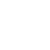Example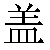SequenceThank you!

WearYourChineseName - By Giuseppe Romanazzi